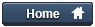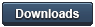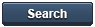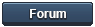### Gammon Forum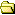Entire forum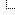Electronics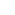Microprocessors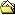Remote controlled car

Remote controlled car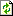Refresh page

Posted by Nick Gammon   Australia  (22,921 posts)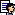bio   Forum Administrator
Date Fri 20 Jan 2012 04:09 AM (UTC)

Amended on Sun 22 Jan 2012 07:23 PM (UTC) by Nick Gammon

Message
 Introduction This post describes a remote-controlled car I have been working on in conjunction with my children. I describe below the various modules used in the final design, in the hope that the various techniques will be useful to others making something similar. Rather than launching into a major circuit description for the whole thing, the individual components will be described, so that you might incorporate bits and pieces into your own designs. Finished vehicle: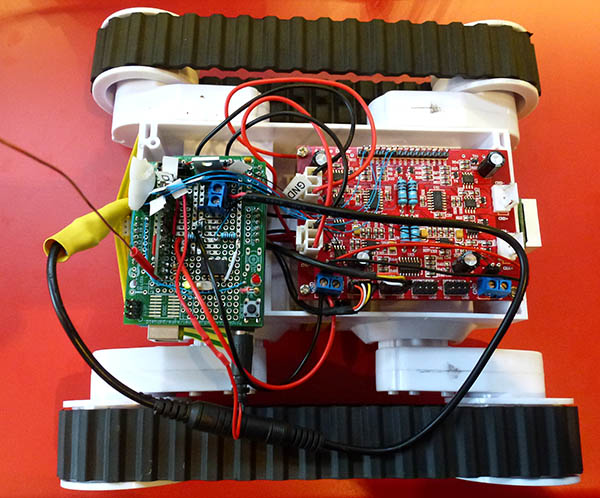Prototyping shield (plugged into Arduino Uno underneath it) with various parts labelled: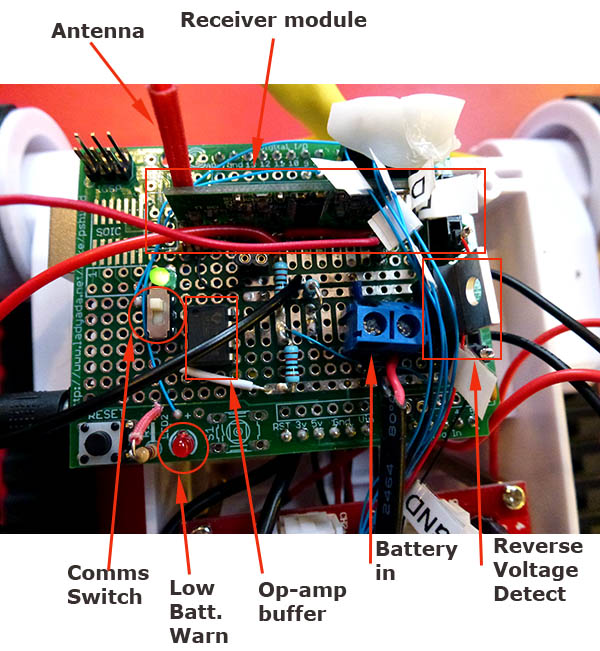Li-Po 7.4V 2S 3300 mAH battery taped to underneath: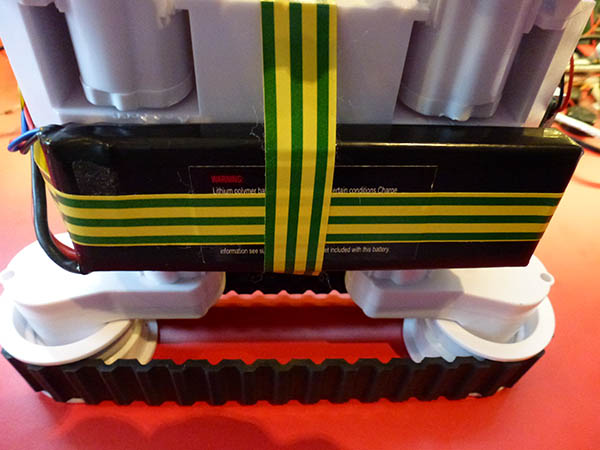Transmitter board (not in a box yet) consisting of Arduino Uno connected to a breadboard with a Wii Nunchuk adapter, and the transmitter module (circled in green):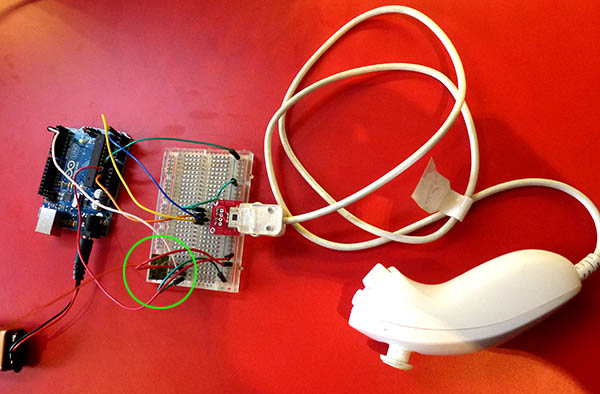Motor controller board To translate the logic level signals into enough power to drive motors we need a controller board, like this one: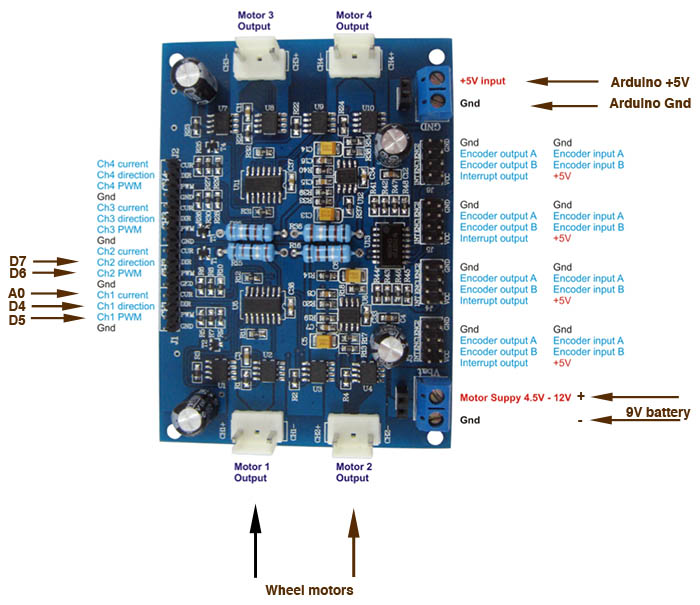The board has "H-Bridge" chips to drive the motors, plus extra logic to detect the amount of current being drawn (by measuring the current flow through the 4 large, blue, "current sense" resistors). This is amplified to a voltage that can be read on an analog port. It also has logic to read the rotary encoders attached to the wheels, and "or" together the encoder outputs so you can get a single interrupt from each one. The interrupt (or encoder) output can be used to detect how fast the wheels are actually turning. Test before assembling ... My advice is to check the transmitter and receiver on a breadboard before trying to solder everything together. Believe me, it's frustrating when you put it all together, and try to test, and nothing happens! It could be: Faulty transmitter Faulty receiver Transmitter and receiver on different frequencies Faulty wiring Coding error (on either of the Arduinos) Wrong length antenna Below I describe how I went about testing both the transmitter and receiver ... Transmitter This is the transmitter board, as you can see it is pretty small: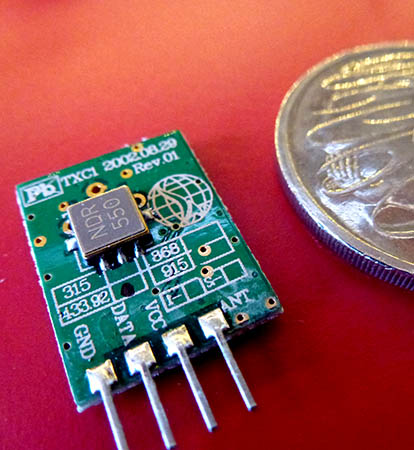Reverse side: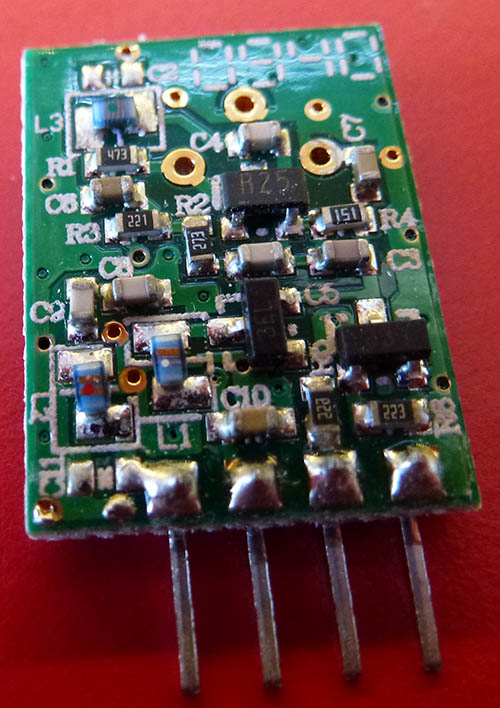It's pretty straightforward to wire it ... you just connect +5V (VCC), Ground, and serial data to the data pin. You can also attach an optional antenna. Details at: `http://www.tato.ind.br/files/TX-C1.pdf` Suggested antenna lengths (from the receiver documentation): About 23 cm for 315 MHz About 17 cm for 434 MHz Receiver The receiver is a bit wider: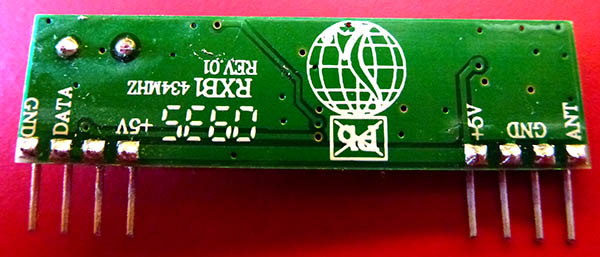Reverse side: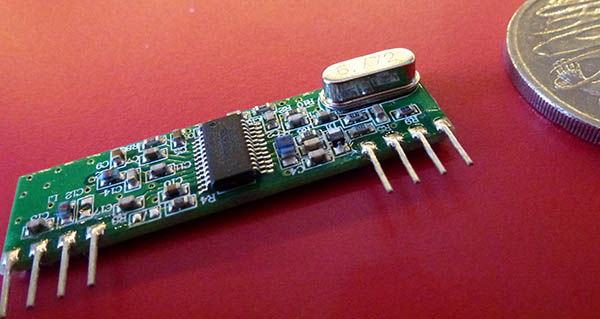Again, you just connect +5V, Ground, and data emerges from the data pin. You can also attach an optional antenna. Test the transmitter On a breadboard, connect up 5V, ground and an antenna. Plug the data pin into the Tx pin (D1) on the Arduino.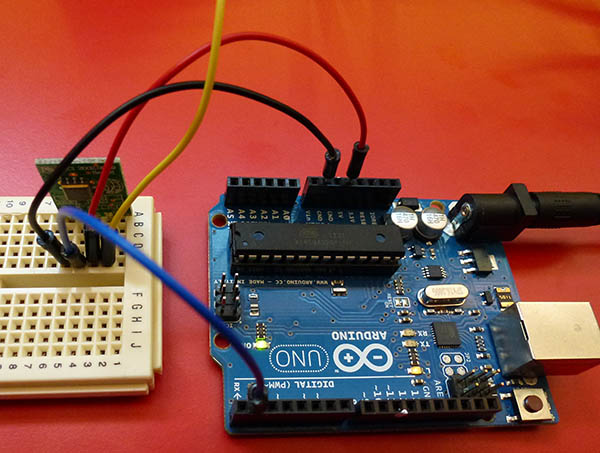The yellow wire is the antenna. This test sketch will continuously output all possible bytes to it: `````` void setup () { Serial.begin (600); } // end of setup void loop () { for (int i = 0; i < 256; i++) Serial.write ((byte) i); } // end of loop `````` Test the receiver On a breadboard, connect up 5V, ground and an antenna.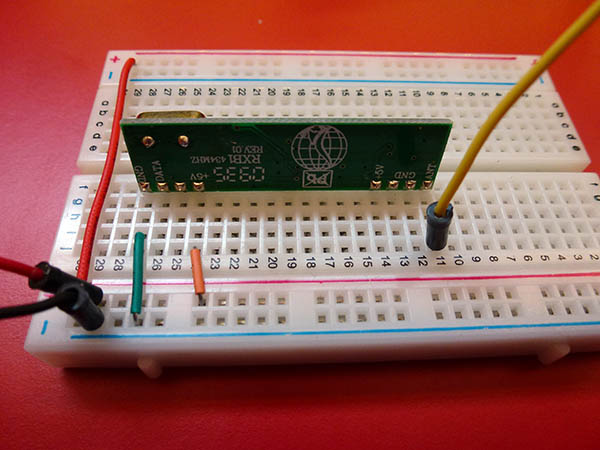We can now hook up an oscilloscope to the data pin and check that something is happening. The yellow line is the data going into the transmitter and the blue one is what we are getting on the receiver...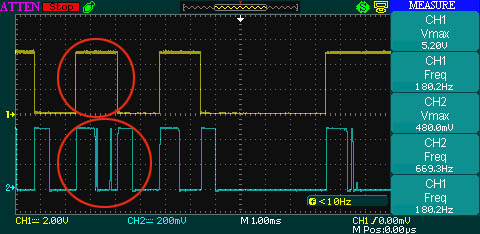Whilst it is nice to see something happening, the receiver isn't getting exactly what the transmitter is sending. I found that by removing the antenna from the transmitter the problem went away. Possibly they were too close to work reliably. Once that was fixed, the results looked like this: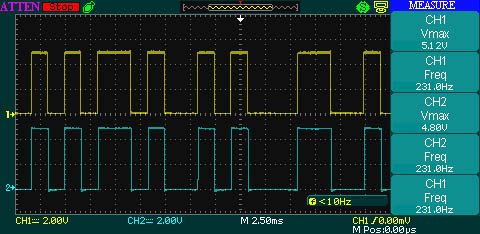Check with logic analyzer It's all very well seeing 0s and 1s, but are they correct? Hooking up the data pin to the logic analyzer shows that we are indeed getting sequential bytes: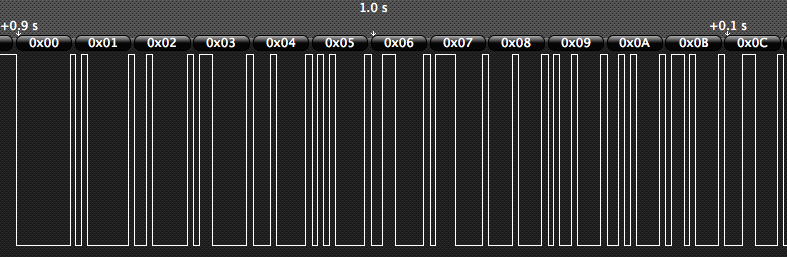Connect receiver to Arduino So, job done, right? We just connect the receiver the the Arduino and we can start sending data. Well, not quite. After connecting the receiver data pin to D0 (the Rx pin) of the Arduino, the oscilloscope suddenly shows this: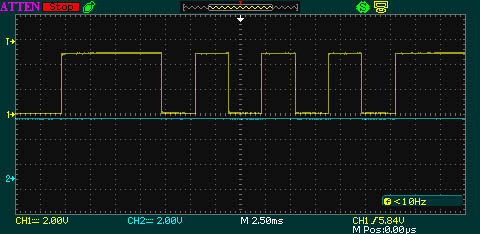It appears that something on the Rx pin is interfering with the data. On a hunch, I connected the receiver up to pin D8, and use the NewSoftSerial library to do a "software serial" read. This time the scope showed this: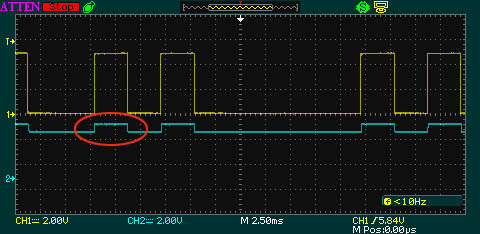It seems that the pull-up resistors used by NewSoftSerial (and also by the USB chip) are "too strong" for the receiver. Operational Amplifier (op-amp) as a buffer So we need to add in a "buffer" chip. I chose to use an LM358 op-amp, and set it up as a comparator. By using two 10K resistors as a voltage divider, the op-amp will output "full voltage" if the incoming voltage is more than 2.5V, and "zero voltage" if it is less than 2.5V.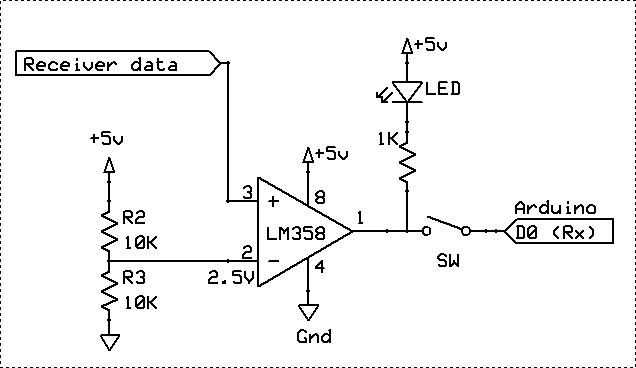Op-amp added to breadboard: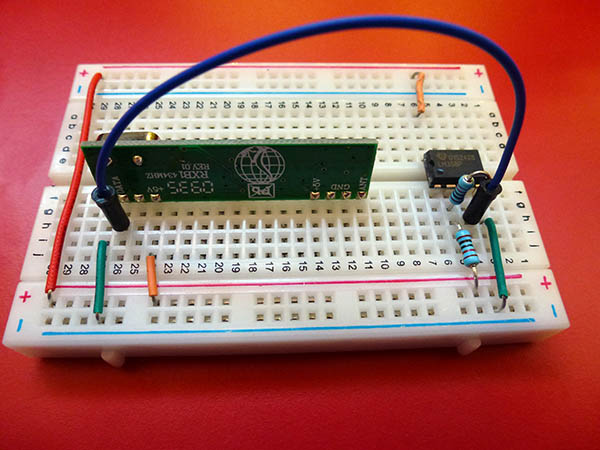And connected to the Arduino: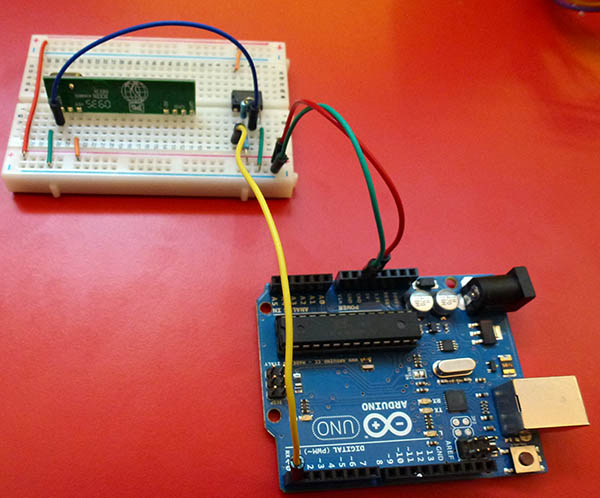Now it all works! In the final version I added a switch between the op-amp and the Arduino Rx (D0) pin - otherwise you can't program the Arduino via the USB port any more, because the op-amp output is too strong for the USB chip. Also, an LED connected between the output and 5V, via a 1K resistor, is designed to light when there is data there. This is because with async serial data is present if the line is low, and there is no data if the line is high. Receiver test sketch To test, we can connect it up to pin D8, and use NewSoftSerial to read from the receiver, and echo back what it got to the serial monitor: ``````#include NewSoftSerial truck (8, 9); // receive pin, transmit pin void setup () { Serial.begin (115200); truck.begin (600); } // end of setup void loop () { if (!truck.available ()) return; byte c = truck.read (); Serial.print (c, HEX); Serial.println (); } // end of loop `````` Error detecting protocol Sending serial data through the air is likely to be fraught with problems (noise, drop-outs) so we need to assure ourselves that the data arrives reliably. There are various ways to do this, I used the "RS485" library I developed for sending serial data via a RS485 link. Details here: http://www.gammon.com.au/forum/?id=11428 Without getting too bogged down at this stage about where the data is coming from, here is a small example of how you can use this library: ``````// Example transmitter #include #include const int WIRELESS_BAUD_RATE = 600; void setup () { Serial.begin (WIRELESS_BAUD_RATE); } // end of setup // callbacks for the sendMsg function void fWrite (const byte what) { Serial.write (what); } // end of fWrite int fAvailable () { return Serial.available (); } // end of fAvailable int fRead () { return Serial.read (); } // end of fRead void loop () { // get data from nunchuk, joystick, switches etc. byte joy_x_axis = 42; byte joy_y_axis = 33; // message for other end byte msg  = { joy_x_axis, joy_y_axis }; // send it sendMsg (fWrite, msg, sizeof msg); } // end of loop `````` The sendMsg function sends the data (in my case, the X and Y position of the nunchuk) to the remote gadget (my wireless car). The function attaches a header (0x02), trailer (0x03) and sumcheck to ensure that the data is correct. The receiver (eg. on the remote-controlled car) just calls recvMsg to get the data sent by the other end. If no message (or the message was corrupted in some way) then we just return, to re-enter the main loop, waiting for another one. ``````// Example receiver #include #include const int WIRELESS_BAUD_RATE = 600; // callback routines void fWrite (const byte what) { Serial.print (what); } // end of fWrite int fAvailable () { return Serial.available (); } // end of fAvailable int fRead () { return Serial.read (); } // end of fRead void setup () { Serial.begin (WIRELESS_BAUD_RATE); } // end of setup void loop () { byte msg ; if (!recvMsg (fAvailable, fRead, msg, sizeof (msg))) return; byte joy_x_axis; byte joy_y_axis; joy_x_axis = msg ; joy_y_axis = msg ; // move car appropriately here } // end of loop `````` Low voltage detector The next issue, which we discovered whilst driving around scaring the cat, was that the battery on the car could get dangerously low. So, a method of detecting low voltage was necessary. Since the car was powered by a Li-Po battery (two cells in series) its valid range was 7.4V to 8.4V. Now 8.4V is too much to plug into an analog port, so we need to get it down to the range 0 to 5V. Thus, a simple voltage divider will do it: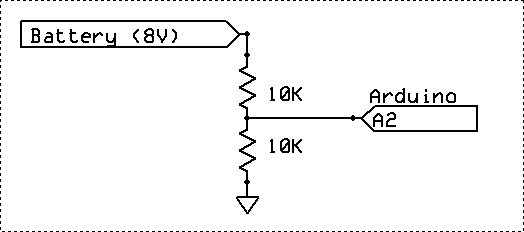Now the voltage on pin A2 will be the battery voltage, halved. So we now need a simple calculation to see if the battery is too low: `````` const float LOW_BATTERY_VOLTAGE = 7.5; const byte BATTERY_CHECK = 2; // A2 // where the motors are connected to const byte LEFT_MOTOR = 5; const byte RIGHT_MOTOR = 6; // warning LED const byte RED_LED = 8; void setup () { // low battery warning LED pinMode (RED_LED, OUTPUT); } // end of setup void loop () { int voltage = analogRead (BATTERY_CHECK); // 1024 would be 5V on the pin // we have a voltage divider so the read voltage is half the battery float volts = voltage / 1024.0 * 5.0 * 2.0; if (volts < LOW_BATTERY_VOLTAGE) { analogWrite (LEFT_MOTOR, 0); // stop motors analogWrite (RIGHT_MOTOR, 0); digitalWrite (RED_LED, HIGH); // flash LED as warning delay (50); digitalWrite (RED_LED, LOW); delay (950); return; // don't try to use motors now } // rest of loop here } // end of loop `````` The above code reads in the voltage from the battery voltage divider. Since it returns 1024 for "full" voltage, then we divide by 1024, multiply by 5 (since full voltage is 5) and then double it to allow for the voltage divider. Then if the resulting voltage is too low (eg. less than 7.5 volts) we turn the motors off, flash the "warning" LED, and exit the loop. Reverse voltage protection Another idea to save a lot of expense is to handle someone plugging the battery in backwards. Afrotechmods did a great tutorial on this: `http://www.youtube.com/watch?v=IrB-FPcv1Dc` The idea here is that, rather than just using a diode (which has a substantial voltage drop of around 0.7V) you use a P-channel MOSFET. The basic circuit is this: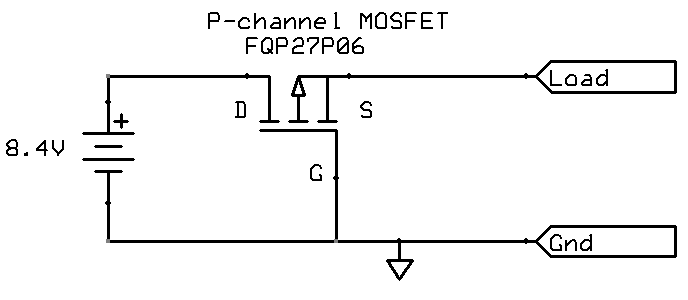As he explains, configured in this way, the MOSFET only allows forward conduction if the battery is plugged in the correct way. In the case of the FQP27P06 transistor, the figure for RDS(on) is typically 0.055 ohms, so at a drain of 5A, the voltage drop would only be 5 * 0.055 = 0.275V. However most diodes have a forward voltage drop of around 0.7V, so that is a much larger voltage drop (and hence, more heat to be dissipated). Of course, with lower current (eg. 2A) then the voltage drop over the MOSFET will be correspondingly lower (eg. 0.11V) compared to the fixed voltage drop of 0.7V with the diode). Overload detection In an earlier version (where the driving was done by an algorithm) I had a "current sense" hooked up. This uses the current sense resistor on the motor board, which has voltage drop over the resistor amplified and sent to analog pin A0. By reading that you can work out how much current is flowing into the motors, and thus if they are working too hard. `````` const byte CURRENTA = 0; // A0 const byte CURRENTB = 1; // A1 const int CURRENT_LIMIT = (1024 / 5) * 2.6; // amps ... if (analogRead (CURRENTA) > CURRENT_LIMIT) { analogWrite (LEFT_MOTOR, 0); // stop motors analogWrite (RIGHT_MOTOR, 0); return; // don't try to use motors now } `````` The example code shows how, in the main loop, you can detect the current being (say) over 2.6 amps, and then shut the motors down if so. The current sense pin is documented to provide 1V per 1A motor drain, thus 5A would be a reading of 1024 on pin A0. Reading the rotary encoders If you want to see how fast the wheels are actually turning, you can connect up the rotary encoder "interrupt" outputs to pins D2 and D3. Then the code snippet below shows how you can count interrupts in an ISR (interrupt service routine). By comparing counts over a time period you can work out what speed the wheels are doing. Or, the ticks themselves just show how far you have gone (of course the wheels might be spinning, so that isn't totally reliable). `````` // rotary encoders - read wheel speed const byte LEFT_ENCODER = 8; const byte RIGHT_ENCODER = 9; const byte INTERRUPTA = 0; // that is, pin 2 const byte INTERRUPTB = 1; // that is, pin 3 volatile unsigned long ticksA; volatile unsigned long ticksB; unsigned long start_timing; int speedA, speedB; // Interrupt Service Routine for a change to encoder pin A void isrA () { ticksA++; } // end of isrA // Interrupt Service Routine for a change to encoder pin B void isrB () { ticksB++; } // end of isrB void setup () { attachInterrupt (INTERRUPTA, isrA, CHANGE); attachInterrupt (INTERRUPTB, isrB, CHANGE); start_timing = millis (); // other setup stuff ... } void loop () { // work out speed over 10th second if (millis () - start_timing > 100) { // grab the data before it changes noInterrupts (); unsigned long interval = millis () - start_timing; speedA = ticksA * 1000L / interval; speedB = ticksB * 1000L / interval; ticksA = ticksB = 0; start_timing = millis (); interrupts (); } // other stuff here ... } // end of loop `````` Emergency Stop One thing that became obvious during testing was that, if something went wrong, the vehicle might power itself away into some dangerous situation, like heading for a flight of stairs. To avoid this we built in a test that, if no data was received from the radio in (say) one second, then the program would stop the motors. `````` const unsigned long TIME_BEFORE_WE_GIVE_UP = 1000; // ms ... byte msg ; if (!recvMsg (fAvailable, fRead, msg, sizeof (msg))) { // no command for a second? stop!!! if (millis () - lastCommand >= TIME_BEFORE_WE_GIVE_UP) { analogWrite (LEFT_MOTOR, 0); // stop motors analogWrite (RIGHT_MOTOR, 0); } return; } // remember when we got a command lastCommand = millis (); `````` This gives us an "out" if the car gets out of range, the transmitter fails, or there is too much radio interference. Transmitter sketch The transmitter program is fairly simple. Below is the complete sketch for it, including the (fairly simple) code to read the various buttons and controls on the Wii Nunchuk: [EDIT] See further down for more up-to-date version which uses Manchester encoding rather than async serial. `````` // Remote controlled vehicle transmitter // Author: Nick Gammon // Date: 14th January 2012 #include #include #include "RS485_protocol.h" const int NUNCHUK_ADDRESS = 0x52; const int WIRELESS_BAUD_RATE = 600; const unsigned long TIME_BEFORE_WE_DEFINITELY_SEND = 250; // ms const unsigned long TIME_BETWEEN_NUNCHUK_READS = 100; // ms const byte LED = 13; void setup () { Serial.begin (WIRELESS_BAUD_RATE); Serial.println (); Wire.begin(); // join i2c bus as master Wire.beginTransmission (NUNCHUK_ADDRESS); Wire.send(0x40); // initialize nunchuk Wire.send(0x00); Wire.endTransmission(); pinMode (LED, OUTPUT); digitalWrite (LED, LOW); } // end of setup unsigned long lastNunchukRead; byte joy_x_axis; byte joy_y_axis; int accel_x_axis; int accel_y_axis; int accel_z_axis; byte z_button; byte c_button; // Encode data to format that most wiimote drivers except // only needed if you use one of the regular wiimote drivers byte nunchuk_decode_byte (byte x) { return (x ^ 0x17) + 0x17; } boolean getNunchukData () { if (millis () - lastNunchukRead < TIME_BETWEEN_NUNCHUK_READS) return false; // too soon Wire.requestFrom (NUNCHUK_ADDRESS, 6);// request data from nunchuk // request next lot Wire.beginTransmission(NUNCHUK_ADDRESS); Wire.send(0); Wire.endTransmission(); // no data from nunchuk if (Wire.available () < 6) return false; joy_x_axis = nunchuk_decode_byte (Wire.receive()); joy_y_axis = nunchuk_decode_byte (Wire.receive()); accel_x_axis = nunchuk_decode_byte (Wire.receive()) * 2; accel_y_axis = nunchuk_decode_byte (Wire.receive()) * 2; accel_z_axis = nunchuk_decode_byte (Wire.receive()) * 2; // the sixth byte contains bits for z and c buttons. // it also contains the least significant bits for the accelerometer data // so we have to check each bit of it byte extra = nunchuk_decode_byte (Wire.receive()); z_button = 0; c_button = 0; // buttons if ((extra >> 0) & 1) z_button = 1; if ((extra >> 1) & 1) c_button = 1; // invert the buttons, so 1 is pressed z_button ^= 1; c_button ^= 1; if ((extra >> 2) & 1) accel_x_axis += 2; if ((extra >> 3) & 1) accel_x_axis += 1; if ((extra >> 4) & 1) accel_y_axis += 2; if ((extra >> 5) & 1) accel_y_axis += 1; if ((extra >> 6) & 1) accel_z_axis += 2; if ((extra >> 7) & 1) accel_z_axis += 1; lastNunchukRead = millis (); return true; } // end of getNunchukData void fWrite (const byte what) { Serial.write (what); } int fAvailable () { return Serial.available (); } int fRead () { return Serial.read (); } // ---------------- MAIN LOOP ------------------- byte old_joy_x_axis; byte old_joy_y_axis; unsigned long lastTransmitTime; void loop () { if (!getNunchukData ()) return; // if we recently sent, and data is the (almost the) same, don't bother if ((old_joy_x_axis & 0xFE) == (joy_x_axis & 0xFE) && (old_joy_y_axis & 0xFE) == (joy_y_axis & 0xFE)) // data same? { int X = map (joy_x_axis, 31, 230, -100, 100); int Y = map (joy_y_axis, 28, 222, -100, 100); if (abs (X) < 5) X = 0; if (abs (Y) < 5) Y = 0; // only a short time elapsed, or sending "stand still" if ((millis () - lastTransmitTime < TIME_BEFORE_WE_DEFINITELY_SEND) || (X == 0 && Y == 0)) // don't keep sending "stand still" return; // no change } byte msg  = {joy_x_axis, joy_y_axis}; digitalWrite (LED, HIGH); sendMsg (fWrite, msg, sizeof msg); digitalWrite (LED, LOW); lastTransmitTime = millis (); old_joy_x_axis = joy_x_axis; old_joy_y_axis = joy_y_axis; } // end of loop `````` To try to minimize the amount of data we are sending at the slow rate of 600 baud the transmitting sketch checks if we are going to send the same thing (give or take the low-order bit) and if so, doesn't send it. However because of the "emergency stop" code on the vehicle, we also make sure we send something every 1/4 of a second, otherwise the car might stop if you held your thumb in the same position for a long time. Vehicle sketch [EDIT] See further down for more up-to-date version which uses Manchester encoding rather than async serial. `````` // Dagu 5 Chassis example. // Author: Nick Gammon // Date: 20th January 2012 #include #include "RS485_protocol.h" const unsigned long TIME_BEFORE_WE_GIVE_UP = 1000; // ms const int WIRELESS_BAUD_RATE = 600; const byte FWD = 0; const byte BACKWD = 1; const float LOW_BATTERY_VOLTAGE = 7.5; // analog pins const byte CURRENTA = 0; // A0 const byte CURRENTB = 1; // A1 const byte BATTERY_CHECK = 2; // A2 // digital pins const byte LEFT_DIRECTION = 4; const byte LEFT_MOTOR = 5; const byte RIGHT_DIRECTION = 7; const byte RIGHT_MOTOR = 6; const byte RED_LED = 8; #define CURRENT_LIMIT (1024 / 5) * 2.6 // amps #define TIME_FORWARDS 10000 #define TIME_BACKWARDS 10000 #define TIME_TURN 1000 #define FULL_SPEED 255 #define SPEED_ADJUST 5 void jog (const byte direction) { digitalWrite (LEFT_DIRECTION, direction); digitalWrite (RIGHT_DIRECTION, direction); analogWrite (LEFT_MOTOR, 128); analogWrite (RIGHT_MOTOR, 128); delay (50); analogWrite (LEFT_MOTOR, 0); analogWrite (RIGHT_MOTOR, 0); delay (50); } // end of jog // callback routines void fWrite (const byte what) { Serial.print (what); } // end of fWrite int fAvailable () { return Serial.available (); } // end of fAvailable int fRead () { return Serial.read (); } // end of fRead void setup () { analogWrite (LEFT_MOTOR, 0); analogWrite (RIGHT_MOTOR, 0); pinMode (LEFT_DIRECTION, OUTPUT); pinMode (RIGHT_DIRECTION, OUTPUT); Serial.begin (WIRELESS_BAUD_RATE); // jog wheels to show we are ready ... jog (FWD); jog (BACKWD); // low battery warning LED pinMode (RED_LED, OUTPUT); } // end of setup // ---------------- MAIN LOOP ------------------- unsigned long lastCommand; void loop () { int voltage = analogRead (BATTERY_CHECK); // 1024 would be 5V on the pin // we have a voltage divider so the read voltage is half the battery float volts = voltage / 1024.0 * 5.0 * 2.0; if (volts < LOW_BATTERY_VOLTAGE) { analogWrite (LEFT_MOTOR, 0); // stop motors analogWrite (RIGHT_MOTOR, 0); digitalWrite (RED_LED, HIGH); // flash LED as warning delay (50); digitalWrite (RED_LED, LOW); delay (950); return; // don't try to use motors now } byte msg ; if (!recvMsg (fAvailable, fRead, msg, sizeof (msg))) { // no command for a second? stop!!! if (millis () - lastCommand >= TIME_BEFORE_WE_GIVE_UP) { analogWrite (LEFT_MOTOR, 0); // stop motors analogWrite (RIGHT_MOTOR, 0); } return; } lastCommand = millis (); byte joy_x_axis = msg ; byte joy_y_axis = msg ; // experimentation shows the Nunchuk goes from about 30 to 230, with around 130 in the middle int X = map (joy_x_axis, 31, 230, -100, 100); int Y = map (joy_y_axis, 28, 222, -100, 100); // ignore slightly off center as that just makes the motor whine if (abs (X) < 5) X = 0; if (abs (Y) < 5) Y = 0; // find hypotenuse float hyp = sqrt (X * X + Y * Y); // find the sine of the angle float s = (float) X / hyp; // find the angle float angle = asin (s) * 100.0; // we want 100 to be 255 on the motor int lspeed = hyp * 2.5; int rspeed = hyp * 2.5; if (Y > abs (X)) // forwards { digitalWrite (LEFT_DIRECTION, FWD); digitalWrite (RIGHT_DIRECTION, FWD); } else if (-Y > abs (X)) // backwards { digitalWrite (LEFT_DIRECTION, BACKWD); digitalWrite (RIGHT_DIRECTION, BACKWD); } else if (X >= abs (Y)) // right { digitalWrite (LEFT_DIRECTION, FWD); digitalWrite (RIGHT_DIRECTION, BACKWD); } else if (-X >= abs (Y)) // left { digitalWrite (LEFT_DIRECTION, BACKWD); digitalWrite (RIGHT_DIRECTION, FWD); } // fiddle around making turning better if (Y > 0) { if (X > 0) { if (angle <= 80) rspeed -= angle * (rspeed * 1.1 / 100); else rspeed = (angle - 80) * (rspeed * 1.1 / 100); } else if (X < 0) { if (abs (angle) <= 80) lspeed -= abs (angle) * (lspeed * 1.1 / 100); else lspeed = (abs (angle) - 80) * (lspeed * 1.1 / 100); } } // end Y > 0 else // Y is negative or zero { if (X > 0) { if (angle <= 80) lspeed -= angle * (lspeed * 1.1 / 100); else lspeed = (angle - 80) * (lspeed * 1.1 / 100); } else if (X < 0) { if (abs (angle) <= 80) rspeed -= abs (angle) * (rspeed * 1.1 / 100); else rspeed = (abs (angle) - 80) * (rspeed * 1.1 / 100); } } // end Y <= 0 // set motor speed, no less than 0 and no greater than 255 analogWrite (LEFT_MOTOR, max (0, min (255, lspeed))); analogWrite (RIGHT_MOTOR, max (0, min (255, rspeed))); } // end of loop `````` - Nick Gammon www.gammon.com.au, www.mushclient.com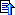top

Posted by Nick Gammon   Australia  (22,921 posts)bio   Forum Administrator
Date Reply #1 on Fri 20 Jan 2012 04:10 AM (UTC)

Amended on Fri 28 Nov 2014 02:03 AM (UTC) by Nick Gammon

Message
 This demonstrates the car in action: (Video cannot be shown because scripting is disabled) - Nick Gammon www.gammon.com.au, www.mushclient.comtop

Posted by Nick Gammon   Australia  (22,921 posts)bio   Forum Administrator
Date Reply #2 on Sun 22 Jan 2012 02:38 AM (UTC)

Amended on Sun 22 Jan 2012 11:00 PM (UTC) by Nick Gammon

Message
 New version with Manchester encoding The remote-controlled car was a bit unreliable for my taste, so I investigated a different way of encoding the data being sent to it. The problem with using Serial comms is that the radio link is not designed to send DC components, but rather changes. And, the gaps between bytes with async serial are just continuous DC. `http://en.wikipedia.org/wiki/Manchester_encoding` The versions below use Manchester encoding instead. This encodes a 0 bit as 10 and a 1 bit as 01. That is, every data bit involves two transitions. I developed two libraries for this purpose ... Manchester sending library http://www.gammon.com.au/Arduino/ManchesterSend.zip This is used to send encoded data (a byte at a time). To do this it users Timer 2 to generate an interrupt exactly every 500 uS. Inside the ISR (interrupt service routine) it clocks out the next pulse. Since each bit requires two pulses this means the transmission rate for data is 1 mS each, or 1000 bits per second. In order for the receiver to distinguish valid data from noise, the sender clocks out 10 "0" bits for synchronization, followed by a single "1" bit. The receiver checks for at least 6 "0" bits, and then waits for a "1" bit. After that it expects 8 data bits. Manchester receiving library http://www.gammon.com.au/Arduino/ManchesterInterrupts.zip This is used to receive the encoded data. I was using a pin-change interrupt on pin D9, as I wanted to keep the normal interrupt pins (D2 and D3) free for the rotary encoders. We turn on the pin-change interrupts like this, in setup: `````` ManchesterInterrupts::dataPin = 9; // pin change interrupts, sigh PCMSK0 = _BV (PCINT1); // only want pin 9 PCIFR = _BV (PCIF0); // clear any outstanding interrupts PCICR |= _BV (PCIE0); // enable pin change interrupts for PCINT0..7 `````` By masking the pin change interrupt we only detect changes on pin 9, and not other pins like 8, 10 and so on. Then we declare the ISR for the pin change interrupt, and call ManchesterInterrupts::isr to actually clock in the bits... `````` // pin change interrupt, do Manchester decoding ISR (PCINT0_vect) { ManchesterInterrupts::isr (); } `````` I wanted to use interrupts so that the car could still drive around, detect obstacles and so on. Once a byte has been detected it is placed into a circular buffer, and then we can detect that the buffer has data in it, and pull that out and process it. Nunchuk library http://www.gammon.com.au/Arduino/Nunchuk.zip To simplify interacting with the Nunchuk, the nunchuk code has been moved into a separate library, which can be downloaded above. Transmitter sketch Amended transmitter sketch below: `````` // Remote controlled vehicle transmitter // Author: Nick Gammon // Date: 14th January 2012 #include #include #include #include const unsigned long TIME_BEFORE_WE_DEFINITELY_SEND = 250; // ms // digital pins const byte SEND_PIN = 8; const byte LED = 13; void setup () { pinMode (LED, OUTPUT); digitalWrite (LED, LOW); ManchesterSend::begin (SEND_PIN); Nunchuk::begin (); } // end of setup // ---------------- MAIN LOOP ------------------- byte old_joy_x_axis; byte old_joy_y_axis; unsigned long lastTransmitTime; void loop () { if (!Nunchuk::read ()) return; // no data // if we recently sent, and data is the (almost the) same, don't bother if ((old_joy_x_axis & 0xFE) == (Nunchuk::joy_x_axis & 0xFE) && (old_joy_y_axis & 0xFE) == (Nunchuk::joy_y_axis & 0xFE)) // data same? { // only a short time elapsed if ((millis () - lastTransmitTime < TIME_BEFORE_WE_DEFINITELY_SEND)) return; // no change } // end if no great change byte msg  = { Nunchuk::joy_x_axis, Nunchuk::joy_y_axis }; digitalWrite (LED, HIGH); sendMsg (ManchesterSend::write, msg, sizeof msg); sendMsg (ManchesterSend::write, msg, sizeof msg); // send again to be sure digitalWrite (LED, LOW); lastTransmitTime = millis (); old_joy_x_axis = Nunchuk::joy_x_axis; old_joy_y_axis = Nunchuk::joy_y_axis; } // end of loop `````` [EDIT] Modified to send codes twice as described further down, also modified to use Nunchuk library. Vehicle sketch Amended vehicle sketch below, major changes in bold: `````` // Dagu Wireless Vehicle Demo // Author: Nick Gammon // Date: 20th January 2012 #include #include const unsigned long TIME_BEFORE_WE_GIVE_UP = 2000; // ms const byte FWD = 0; const byte BACKWD = 1; const float LOW_BATTERY_VOLTAGE = 7.5; // analog pins const byte CURRENTA = 0; // A0 const byte CURRENTB = 1; // A1 const byte BATTERY_CHECK = 2; // A2 // digital pins const byte LEFT_DIRECTION = 4; const byte LEFT_MOTOR = 5; const byte RIGHT_DIRECTION = 7; const byte RIGHT_MOTOR = 6; const byte RED_LED = 8; const byte DATA_PIN = 9; void jog (const byte direction) { digitalWrite (LEFT_DIRECTION, direction); digitalWrite (RIGHT_DIRECTION, direction); analogWrite (LEFT_MOTOR, 128); analogWrite (RIGHT_MOTOR, 128); delay (50); analogWrite (LEFT_MOTOR, 0); analogWrite (RIGHT_MOTOR, 0); delay (50); } // end of jog // pin change interrupt, do Manchester decoding ISR (PCINT0_vect) { ManchesterInterrupts::isr (); } // end of PCINT0_vect void setup () { analogWrite (LEFT_MOTOR, 0); analogWrite (RIGHT_MOTOR, 0); pinMode (LEFT_DIRECTION, OUTPUT); pinMode (RIGHT_DIRECTION, OUTPUT); ManchesterInterrupts::dataPin = DATA_PIN; // pin change interrupts, sigh PCMSK0 = _BV (PCINT1); // only want pin 9 PCIFR = _BV (PCIF0); // clear any outstanding interrupts PCICR |= _BV (PCIE0); // enable pin change interrupts for PCINT0..7 // jog wheels to show we are ready ... jog (FWD); jog (BACKWD); // low battery warning LED pinMode (RED_LED, OUTPUT); } // end of setup // ---------------- MAIN LOOP ------------------- unsigned long lastCommand; void loop () { int voltage = analogRead (BATTERY_CHECK); // 1024 would be 5V on the pin // we have a voltage divider so the read voltage is half the battery float volts = voltage / 1024.0 * 5.0 * 2.0; if (volts < LOW_BATTERY_VOLTAGE) { analogWrite (LEFT_MOTOR, 0); // stop motors analogWrite (RIGHT_MOTOR, 0); digitalWrite (RED_LED, HIGH); // flash LED as warning delay (50); digitalWrite (RED_LED, LOW); delay (950); return; // don't try to use motors now } byte msg ; if (!recvMsg (ManchesterInterrupts::available, ManchesterInterrupts::read, msg, sizeof (msg))) { // no command for a second? stop!!! if (millis () - lastCommand >= TIME_BEFORE_WE_GIVE_UP) { analogWrite (LEFT_MOTOR, 0); // stop motors analogWrite (RIGHT_MOTOR, 0); } // end of stopping the motors return; } // end of no command received lastCommand = millis (); byte joy_x_axis = msg ; byte joy_y_axis = msg ; // experimentation shows the Nunchuk goes from about 30 to 230, with around 130 in the middle int X = map (joy_x_axis, 31, 230, -100, 100); int Y = map (joy_y_axis, 28, 222, -100, 100); // ignore slightly off center as that just makes the motor whine if (abs (X) < 5) X = 0; if (abs (Y) < 5) Y = 0; // find hypotenuse float hyp = sqrt (X * X + Y * Y); // find the sine of the angle float s = (float) X / hyp; // find the angle float angle = asin (s) * 100.0; // we want 100 to be 255 on the motor int lspeed = hyp * 2.5; int rspeed = hyp * 2.5; if (Y > abs (X)) // forwards { digitalWrite (LEFT_DIRECTION, FWD); digitalWrite (RIGHT_DIRECTION, FWD); } else if (-Y > abs (X)) // backwards { digitalWrite (LEFT_DIRECTION, BACKWD); digitalWrite (RIGHT_DIRECTION, BACKWD); } else if (X >= abs (Y)) // right { digitalWrite (LEFT_DIRECTION, FWD); digitalWrite (RIGHT_DIRECTION, BACKWD); } else if (-X >= abs (Y)) // left { digitalWrite (LEFT_DIRECTION, BACKWD); digitalWrite (RIGHT_DIRECTION, FWD); } // fiddle around making turning better if (Y > 0) { if (X > 0) { if (angle <= 80) rspeed -= angle * (rspeed * 1.1 / 100); else rspeed = (angle - 80) * (rspeed * 1.1 / 100); } // X <= 0 else { if (abs (angle) <= 80) lspeed -= abs (angle) * (lspeed * 1.1 / 100); else lspeed = (abs (angle) - 80) * (lspeed * 1.1 / 100); } } // end Y > 0 else // Y <= 0 { if (X > 0) { if (angle <= 80) lspeed -= angle * (lspeed * 1.1 / 100); else lspeed = (angle - 80) * (lspeed * 1.1 / 100); } // X <= 0 else { if (abs (angle) <= 80) rspeed -= abs (angle) * (rspeed * 1.1 / 100); else rspeed = (abs (angle) - 80) * (rspeed * 1.1 / 100); } } // end Y <= 0 // set motor speed, no less than 0 and no greater than 255 analogWrite (LEFT_MOTOR, max (0, min (255, lspeed))); analogWrite (RIGHT_MOTOR, max (0, min (255, rspeed))); } // end of loop `````` - Nick Gammon www.gammon.com.au, www.mushclient.comtop

Posted by Nick Gammon   Australia  (22,921 posts)bio   Forum Administrator
Date Reply #3 on Sun 22 Jan 2012 07:07 PM (UTC)

Amended on Sun 22 Jan 2012 07:55 PM (UTC) by Nick Gammon

Message
 Another technique for improved reliability After reading about the way infra-red TV remotes work, I got an idea for making the car more reliable. Apparently TV remotes often send the same codes twice, in the hope that at least one of them would get received. So, in the "transmitter" sketches above (whichever one you use) replace: `````` sendMsg (fWrite, msg, sizeof msg); `````` by: `````` sendMsg (fWrite, msg, sizeof msg); sendMsg (fWrite, msg, sizeof msg); // send again `````` It won't hurt the vehicle to get the same message twice, and hopefully one at least will get through. This seems to make it more responsive. (Note, second transmitter sketch - the one using Manchester encoding - has been modified to do the send twice). - Nick Gammon www.gammon.com.au, www.mushclient.comtop

The dates and times for posts above are shown in Universal Co-ordinated Time (UTC).

To show them in your local time you can join the forum, and then set the 'time correction' field in your profile to the number of hours difference between your location and UTC time.

32,643 views.Refresh page

Go to topic:           Search the forumtop

Quick links: MUSHclient. MUSHclient help. Forum shortcuts. Posting templates. Lua modules. Lua documentation.

Information and images on this site are licensed under the Creative Commons Attribution 3.0 Australia License unless stated otherwise.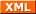Forum RSS feed ( https://gammon.com.au/rss/forum.xml )